Function Repository Resource:

# IndexedSubset

Give the index of a subset or return the subset with that index

Contributed by: Ed Pegg Jr
 ResourceFunction["IndexedSubset"][choose,index] returns a subset of length choose with given index. ResourceFunction["IndexedSubset"][list] gives the index of subset list.

## Details and Options

For any positive integers n and k, n can be uniquely represented as k binomials,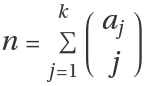, where the aj terms are strictly increasing.

## Examples

### Basic Examples (2)

The following 3-subset ordering can be extended to infinity:

 In:=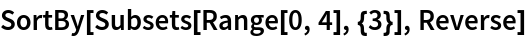Out=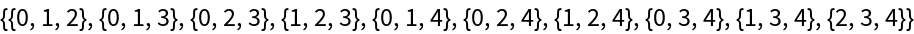The function returns the same subsets in the same order:

 In:=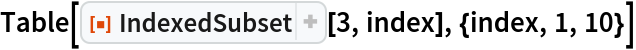Out=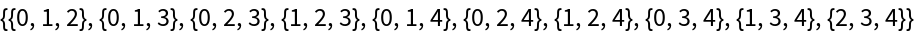The function can return subsets of a large index:

 In:=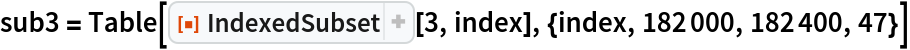Out=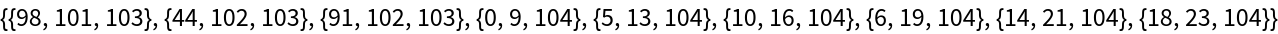Applying the function to a subset returns the index:

 In:=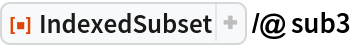Out=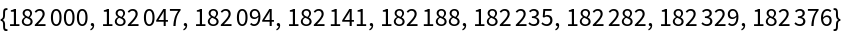Any strictly increasing list of integers can be considered as a subset with a unique index:

 In:=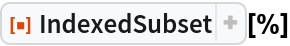Out=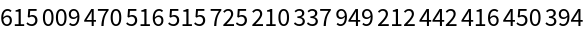The index above generates a unique 9-subset:

 In:=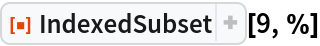Out=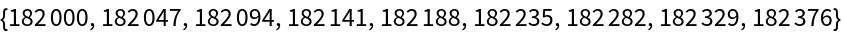### Scope (4)

Some binomial representations of the number 320:

 In:=Out=Here are some two item subsets with their indices to show their structure:

 In:=Out=The 2-subset {4,5} has an index of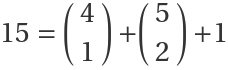:

 In:=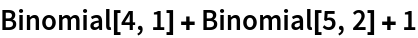Out=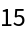Recover the same information using IndexedSubset:

 In:=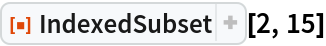Out=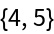The structure of 3-subsets in 3D:

 In:=Out=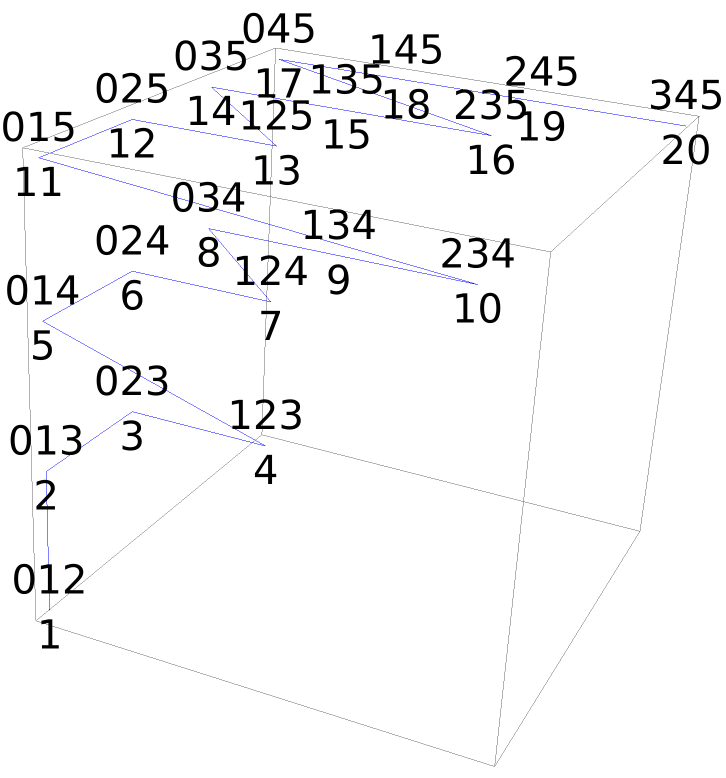### Neat Examples (2)

Find the trillionth number with binary weight eight:

 In:=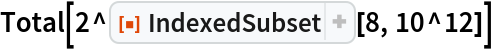Out=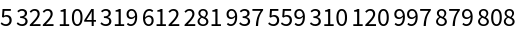Find the index of an eight-term subset:

 In:=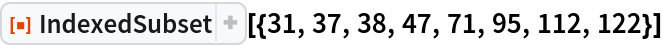Out=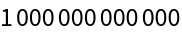## Version History

• 1.0.0 – 06 November 2019